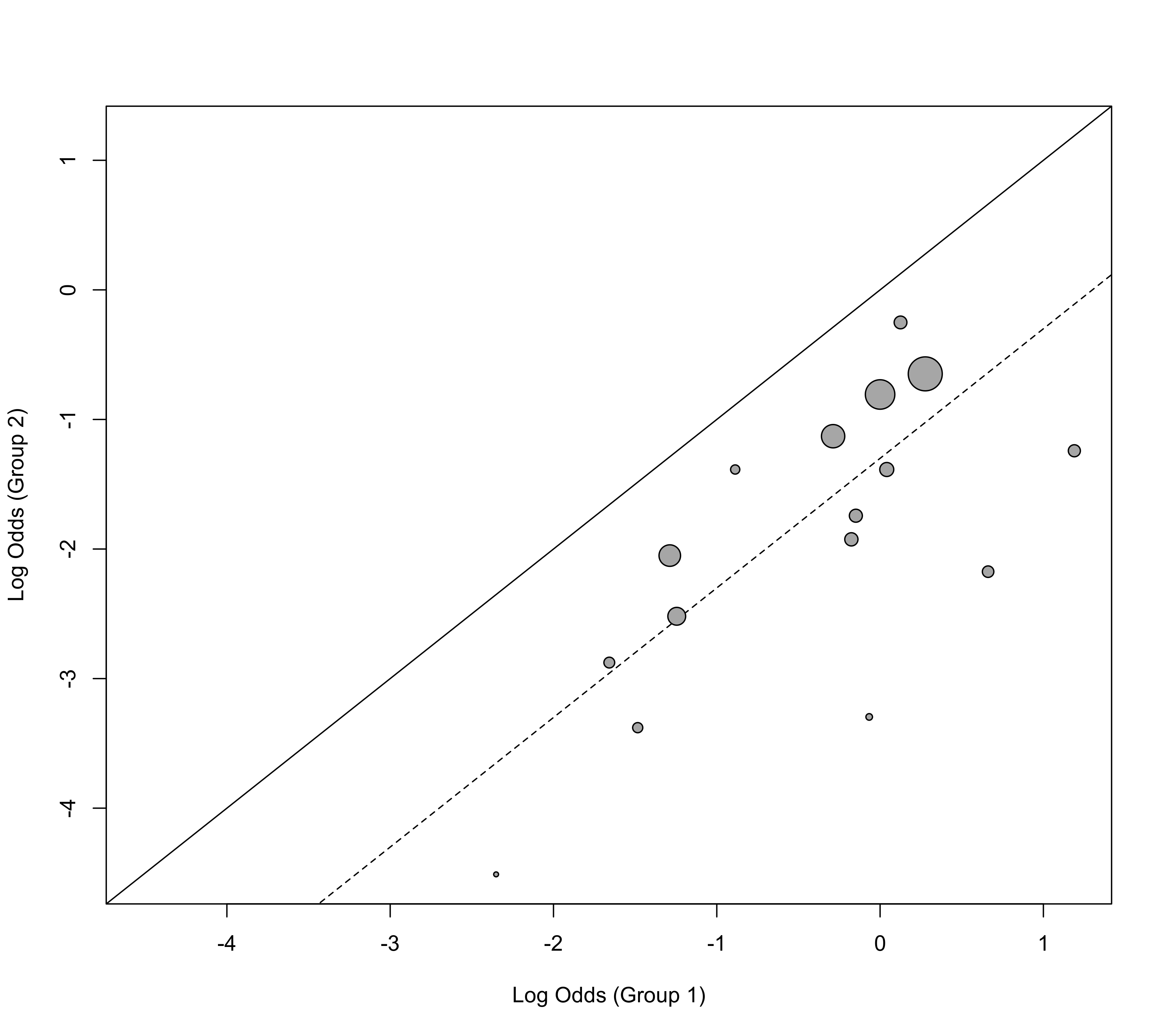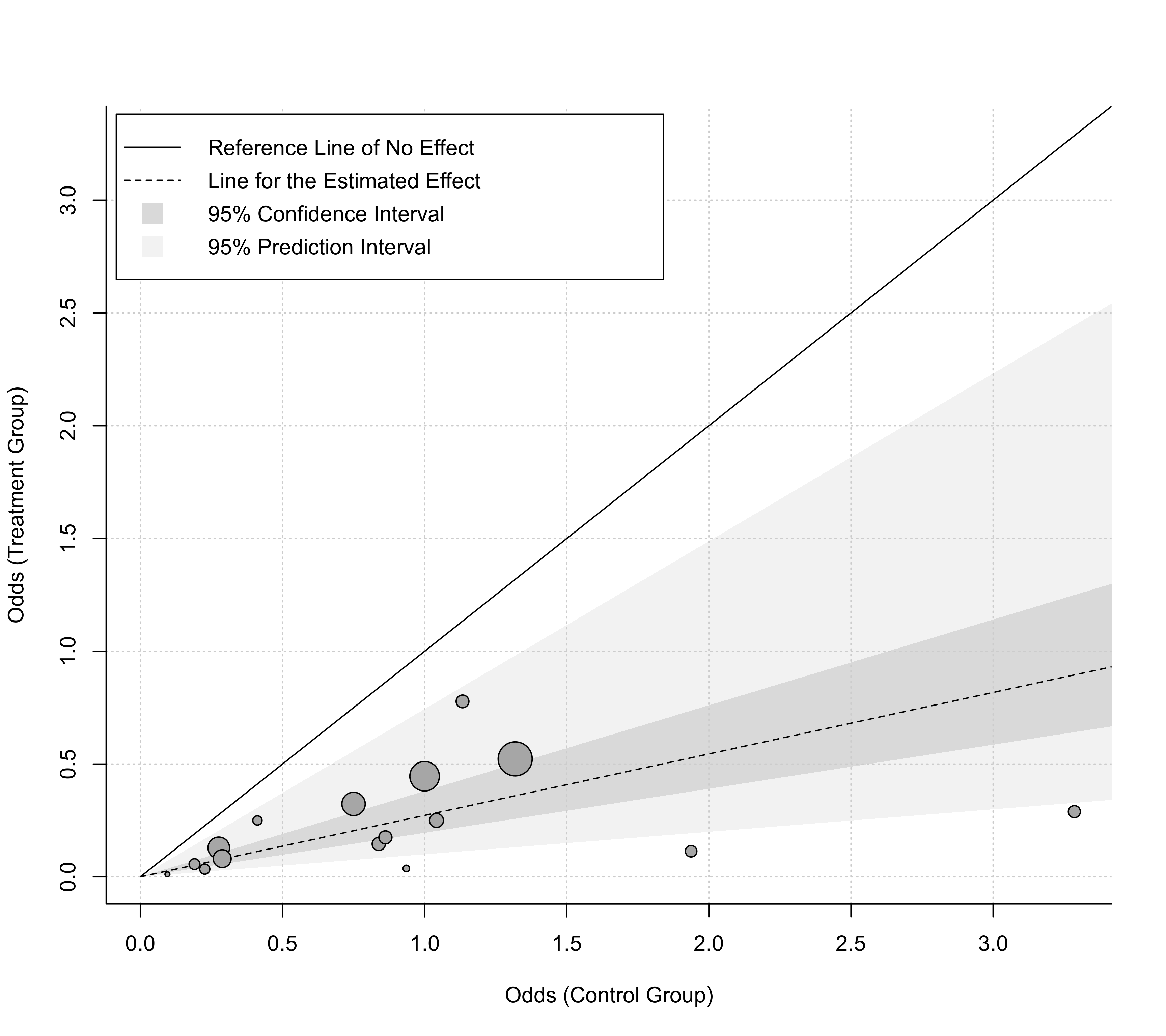Function to create L'Abbé plots for objects of class "rma".

labbe(x, ...)

# S3 method for rma
labbe(x, xlim, ylim, xlab, ylab,
add=x$add, to=x$to, transf, targs,
pch=21, psize, plim=c(0.5,3.5),
col, bg, grid=FALSE, lty, ...)

## Arguments

x

an object of class "rma".

xlim

x-axis limits. If unspecified, the function sets the x-axis limits to some sensible values.

ylim

y-axis limits. If unspecified, the function sets the y-axis limits to some sensible values.

xlab

title for the x-axis. If unspecified, the function sets an appropriate axis title.

ylab

title for the y-axis. If unspecified, the function sets an appropriate axis title.

See the documentation of the escalc function for more details.

to

See the documentation of the escalc function for more details.

transf

optional argument to specify a function to transform the outcomes (e.g., transf=exp; see also transf). If unspecified, no transformation is used.

targs

optional arguments needed by the function specified under transf.

pch

plotting symbol to use for the outcomes. By default, an open circle is used. Can also be a vector of values. See points for other options.

psize

optional numeric vector to specify the point sizes for the outcomes. If unspecified, the point sizes are a function of the precision of the outcomes. Can also be a vector of values.

plim

numeric vector of length 2 to scale the point sizes (ignored when psize is specified). See ‘Details’.

col

optional character string to specify the (border) color of the points. Can also be a vector.

bg

optional character string to specify the background color of open plot symbols. Can also be a vector. Set to NA to make the plotting symbols transparent.

grid

logical to specify whether a grid should be added to the plot. Can also be a color name.

lty

optional character vector to specify the line type for the diagonal reference line of no effect and the line that indicates the estimated effect based on the fitted model. If unspecified, the function sets this to c("solid","dashed") by default (use "blank" to suppress a line).

...

other arguments.

## Details

The model specified via x must be a model without moderators (i.e., either an equal- or a random-effects model) fitted with either the rma.uni, rma.mh, rma.peto, or rma.glmm functions. Moreover, the model must have been fitted with measure set equal to "RD" (for risk differences), "RR" (for risk ratios), "OR" (for odds ratios), "AS" (for arcsine square root transformed risk differences), "IRR" (for incidence rate ratios), "IRD" (for incidence rate differences), or "IRSD" (for square root transformed incidence rate differences).

The function calculates the arm-level outcomes for the two groups (e.g., treatment and control) and plots them against each other. In particular, the function plots the raw proportions of the two groups against each other when analyzing risk differences, the log of the proportions when analyzing (log) risk ratios, the log odds when analyzing (log) odds ratios, the arcsine square root transformed proportions when analyzing arcsine square root transformed risk differences, the raw incidence rates when analyzing incidence rate differences, the log of the incidence rates when analyzing (log) incidence rate ratios, and the square root transformed incidence rates when analyzing square root transformed incidence rate differences. The transf argument can be used to transform these values (e.g., transf=exp to transform the log of the proportions back to raw proportions; see also transf).

As described under the documentation for the escalc function, zero cells can lead to problems when calculating particular outcomes. Adding a small constant to the cells of the $$2 \times 2$$ tables is a common solution to this problem. By default, the functions adopts the same method for handling zero cells as was used when fitting the model.

By default (i.e., when psize is not specified), the point sizes are a function of the precision (i.e., inverse standard errors) of the outcomes. This way, more precise estimates are visually more prominent in the plot. By making the point sizes a function of the inverse standard errors of the estimates, their areas are proportional to the inverse sampling variances, which corresponds to the weights they would receive in an equal-effects model. However, the point sizes are rescaled so that the smallest point size is plim and the largest point size is plim. As a result, their relative sizes (i.e., areas) no longer exactly correspond to their relative weights in such a model. If exactly relative point sizes are desired, one can set plim to NA, in which case the points are rescaled so that the smallest point size corresponds to plim and all other points are scaled accordingly. As a result, the largest point may be very large. Alternatively, one can set plim to NA, in which case the points are rescaled so that the largest point size corresponds to plim and all other points are scaled accordingly.

The solid line corresponds to identical outcomes in the two groups (i.e., the absence of a difference between the two groups). The dashed line indicates the estimated effect based on the fitted model.

## Value

A data frame with components:

x

the x-axis coordinates of the points that were plotted.

y

the y-axis coordinates of the points that were plotted.

cex

the point sizes.

pch

the plotting symbols.

col

the point colors.

bg

the background colors.

ids

the study id numbers.

slab

the study labels.

Note that the data frame is returned invisibly.

## Author

Wolfgang Viechtbauer wvb@metafor-project.org https://www.metafor-project.org

rma.uni, rma.mh, rma.peto, and rma.glmm for functions to fit models for which L'Abbé plots can be drawn.

## Examples

### meta-analysis of the log risk ratios using a random-effects model
res <- rma(measure="RR", ai=tpos, bi=tneg, ci=cpos, di=cneg, data=dat.bcg)

### default plot
labbe(res)### funnel plot with risk values on the x- and y-axis and add grid
labbe(res, transf=exp, grid=TRUE)